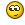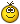#### Тема: Програма виконується в GCC і вилітає у Visual Studio

Завдання: Дано вираз без дужок, у якому зустрічаються операції +, -,* та /. Обчислити значення виразу. Наприклад, для виразу 11 * 2  +  5 * 3 повинні отримати 37. Використати бібліотечні функції на вибір atoi() , atof(),strpbrk(), strcspn() strtod() strtol().

Реалізувала так:

Прихований текст
``````#include <iostream>
#include <cstring>
#include <cstdlib> // this include is not needed for Visual Studio

using namespace std;

/** Gets input from keyboard
*
*  @return - char *expr
*/
char *input_expression()
{
cout << "Enter expression: ";
char *expr = new char;
cin.getline(expr, 256);
return expr;
}

/** Deletes element from array
*
* @param arr - array of any type
* @param size - size of array arr
* @param pos - index of element to delete
*/
template <class T>
void delete_element(T *arr, int &size, int pos)
{
for (int i = pos; i < size; i++)
arr[i]=arr[i+1];
--size;
}

/** Gets intermediate result and makes appropriate changes in expression
* which represented by array of numbers and array of operations.
*
* Example: 4 + 2 * 3  => numbers=(4, 2, 3), operations=(+, *)
* After applaying mid result: numbers=(4, 6), operations=(+,)
*
* @param operations - array of operations in expression
* @param numbers - array of numbers in expression
* @param op_counter - size of array operations
* @param num_counter - size of array num_counter
* @param i - index of changed elements
* @param mid_result - result of calculation of current operation
*/
void apply_mid_result(char *operations, double *numbers,
int op_counter, int num_counter,
int i, double mid_result)
{
delete_element(operations, op_counter, i);
delete_element(numbers, num_counter, i);
numbers[i] = mid_result;
}

/** Parses given expression as string to arrays of numbers and operations.
* Calculates according to priority of operations, returns result of calculation.
*
* NOTE: can calculate expressions without brackets and with standard
* arithmetic operations - addition, subtraction, multiplication, division.
*
* @param expr - arithmetic expression, stored in array of char,
* @return double result
*/
double calculate(char *expr)
{
// get all operations
char operations;
int op_counter = 0;
char oper_signs[] = "*/+-";

char *pch = expr;
pch = strpbrk(pch, oper_signs);
while (pch != NULL)
{
operations[op_counter] = *pch;
pch = strpbrk(pch+1, oper_signs);
++op_counter;
};
cout << "\n";

// get all numbers
double numbers;
int num_counter = 0;
double number;

pch = expr;
while (*pch != '\0')
{
number = strtod(pch, &pch);
numbers[num_counter] = number;
++num_counter;
pch += strcspn(pch, oper_signs) + 1;
}

// make calculation
double result = 0, mid_result;
int i = 0;

// first - high priority operations
while (i < op_counter)
{
if (operations[i] == '*')
{
mid_result = numbers[i] * numbers[i+1];
apply_mid_result(operations, numbers, op_counter, num_counter, i, mid_result);
}
else if (operations[i] == '/')
{
mid_result = numbers[i] / numbers[i+1];
apply_mid_result(operations, numbers, op_counter, num_counter, i, mid_result);
}
else ++i;
}

// last - low priority operations
i = 0;
while (i < op_counter)
{
if (operations[i] == '+')
{
mid_result = numbers[i] + numbers[i+1];
apply_mid_result(operations, numbers, op_counter, num_counter, i, mid_result);
}
else if (operations[i] == '-')
{
mid_result = numbers[i] - numbers[i+1];
apply_mid_result(operations, numbers, op_counter, num_counter, i, mid_result);
}
else ++i;
}

return mid_result;
}

int main()
{
char *expr = input_expression();
cout << "\nExpression: " << expr;
double result = calculate(expr);
cout << "Result: " << result;
return 0;
}``````

GCC(g++) скомпілював і все працює, але при спробі перенести цей код у Visual Studio програма вилітає на етапі виведення результату. В режимі відлагодження все порахувало нормально, вивело результат - нічого поганого не помітила.
В чому може бути проблема?
P.S. Чи можна покращити код?

#### Re: Програма виконується в GCC і вилітає у Visual Studio

Перевірте чи консольну ви програму створюєте в VS (в командному рядку для компілера мусить бути аргумент /SUBSYSTEM:CONSOLE)

#### Re: Програма виконується в GCC і вилітає у Visual Studio

``````    char *expr = new char;
cin.getline(expr, 256);``````

Виділяєте 1 символ і записуєте туди 256...

В цілому ж:
1. Програма ніби на C++, але з рядками і масивами працює, ніби це голий C. std::vector і std::string сильно полегшують життя. Так, дурнувата умова, але це виглядає як їздити на мотоциклі, відштовхуючись ногами від землі.
2. Немає унарних операцій; -1*-1 - цілком відповідає умові і має сенс, але викличе збій програми.
3. Немає перевірки на коректність коду.
В цілому я б радив скористатися або рекурсією, або ПОЛІЗом для кращого узагальнення роботи програми.

#### Re: Програма виконується в GCC і вилітає у Visual Studio

Перевірте чи консольну ви програму створюєте в VS (в командному рядку для компілера мусить бути аргумент /SUBSYSTEM:CONSOLE)

Так, програма консольна

koala написав:
``````        char *expr = new char;
cin.getline(expr, 256);``````

Виділяєте 1 символ і записуєте туди 256...

В цілому ж:
1. Програма ніби на C++, але з рядками і масивами працює, ніби це голий C. std::vector і std::string сильно полегшують життя. Так, дурнувата умова, але це виглядає як їздити на мотоциклі, відштовхуючись ногами від землі.
2. Немає унарних операцій; -1*-1 - цілком відповідає умові і має сенс, але викличе збій програми.
3. Немає перевірки на коректність коду.
В цілому я б радив скористатися або рекурсією, або ПОЛІЗом для кращого узагальнення роботи програми.

0. Додала квадратні дужки до new char - не допомогло.
1. Таке завдання - не я придумала, мені лише потрібно придумати як її реалізувати.
2. Над цим попрацюю - вилетіло геть з голови3. Що саме перевірити? Вхідний рядок?
4. Що таке ПОЛІЗ? Ми, скоріш за все, його ще не вивчали.

#### Re: Програма виконується в GCC і вилітає у Visual Studio

0. так додавали? Я не телепат же.

``char *expr = new char; ``

3. Так, очевидно.
4. https://uk.wikipedia.org/wiki/Польський_інверсний_запис - краще не чекайте, коли будете вивчати, а вивчайте одразуПодякували: 2

#### Re: Програма виконується в GCC і вилітає у Visual Studio

Ось виправила щодо від'ємних чисел:

Прихований текст
``````#include <iostream>
#include <cstring>
#include <cstdlib>

using namespace std;

/** Gets input from keyboard
*
*  @return - char *expr
*/
char *input_expression()
{
cout << "Enter expression: ";
char *expr = new char;
cin.getline(expr, 256);
return expr;
}

/** Deletes element from array
*
* @param arr - array of any type
* @param size - size of array arr
* @param pos - index of element to delete
*/
template <class T>
void delete_element(T *arr, int &size, int pos)
{
for (int i = pos; i < size; i++)
arr[i]=arr[i+1];
--size;
}

/** Gets intermediate result and makes appropriate changes in expression
* which represented by array of numbers and array of operations.
*
* Example: 4 + 2 * 3  => numbers=(4, 2, 3), operations=(+, *)
* After applaying mid result: numbers=(4, 6), operations=(+,)
*
* @param operations - array of operations in expression
* @param numbers - array of numbers in expression
* @param op_counter - size of array operations
* @param num_counter - size of array num_counter
* @param i - index of changed elements
* @param mid_result - result of calculation of current operation
*/
void apply_mid_result(char *operations, double *numbers,
int op_counter, int num_counter,
int i, double mid_result)
{
delete_element(operations, op_counter, i);
delete_element(numbers, num_counter, i);
numbers[i] = mid_result;
}

/** Parses given expression as string to arrays of numbers and operations.
*
* @param expr - arithmetic expression, stored in array of char
* @param numbers - array of double, used as storage of all operands
* @param num_counter - size of array numbers
* @param operations - array of char, used as storage of all operations
* @param op_counter - size of array operations
*/
void parse_expression(char *expr, double *numbers, int &num_counter,
char *operations, int &op_counter)
{
char oper_signs[] = "*/+-";
double number;
char *pch = expr;
while (pch != NULL)
{
number = strtod(pch, &pch);
numbers[num_counter] = number;
++num_counter;
pch = strpbrk(pch, oper_signs);
if (pch != NULL)
{
operations[op_counter] = *pch;
++op_counter;
++pch;
}
}
}

/** Calculates according to priority of operations, returns result of calculation.
*
* NOTE: can calculate expressions without brackets and with standard
* arithmetic operations - addition, subtraction, multiplication, division.
*
* @param expr - arithmetic expression, stored in array of char
* @return double result
*/
double calculate(char *expr)
{
char operations;
int op_counter = 0;

double numbers;
int num_counter = 0;

parse_expression(expr, numbers, num_counter, operations, op_counter);

double result = 0, mid_result;
int i = 0;

// first - high priority operations
while (i < op_counter)
{
if (operations[i] == '*')
{
mid_result = numbers[i] * numbers[i+1];
apply_mid_result(operations, numbers, op_counter, num_counter, i, mid_result);
}
else if (operations[i] == '/')
{
mid_result = numbers[i] / numbers[i+1];
apply_mid_result(operations, numbers, op_counter, num_counter, i, mid_result);
}
else ++i;
}

// last - low priority operations
i = 0;
while (i < op_counter)
{
if (operations[i] == '+')
{
mid_result = numbers[i] + numbers[i+1];
apply_mid_result(operations, numbers, op_counter, num_counter, i, mid_result);
}
else if (operations[i] == '-')
{
mid_result = numbers[i] - numbers[i+1];
apply_mid_result(operations, numbers, op_counter, num_counter, i, mid_result);
}
else ++i;
}

return mid_result;
}

int main()
{
char *expr = input_expression();
cout << "\nExpression: " << expr;
double result = calculate(expr);
cout << "\nResult: " << result << "\n";
return 0;
}``````

1. Підкажіть, будь ласка, як правильно виконати перевірку на коректність.
2. Як можна покращити код у функції calculate?